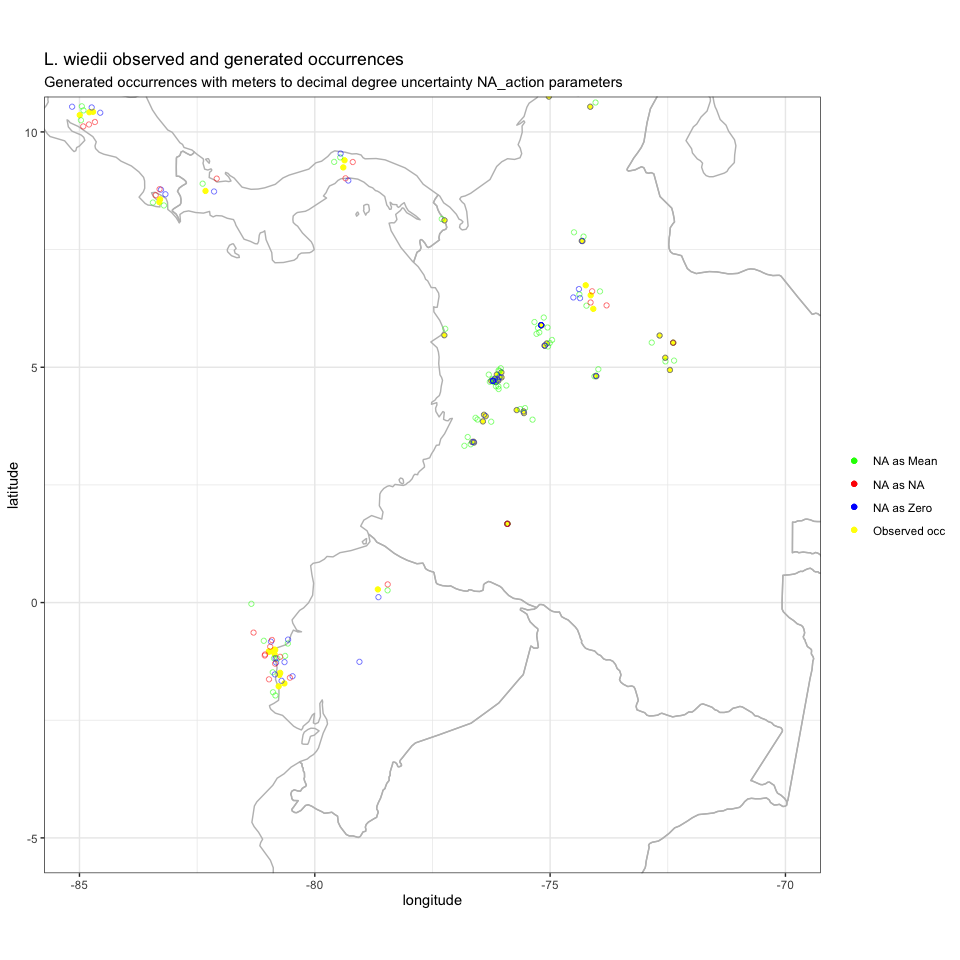# 1. Introduction

This vignette goes through the use of uncertainty measures of species occurrence records in “occUncertainty: An R package for addressing uncertainty of species occurrence in species distribution”. Here we demonstrate how occUncertain can be used to transform uncertainty measures from meters to decimal degrees. Perform iterations to assess occurrence records variability in the quantification of the extent area that encompass the inferred or projected sites of occurrence of a species, and the area within the extent polygon they occupy.

## Input file

To demonstrate the use of the package occUncertain we used a set of 229 verified, georeferenced occurrence records for the margay (Leopardus weidii) a small wild cat native to Central and South America. These occurrences data set are from the Global Biodiversity Information Facility (GBIF) and are included as part of the occUncertain package.

The data set requires to have at least four columns:

1. Latitude in decimal degrees
2. Longitude in decimal degrees
3. Coordinate uncertainty in meters.
4. Name of taxa
latitude longitude coordinateUncertaintyInMeters species
-12.66078 -68.75897 31069 Leopardus wiedii
-30.39700 -51.45458 NA Leopardus wiedii
-30.22583 -54.75972 NA Leopardus wiedii
-30.22440 -51.40551 NA Leopardus wiedii
-30.20297 -54.14224 NA Leopardus wiedii
-28.93862 -53.35768 29558 Leopardus wiedii

# 2. meters_to_decdeg function

The function meters_to_decdeg transforms uncertainty from meters into decimal degrees for each occurrence point.

**Note 1: Is important to consider the fact that as one moves away from the equator towards a pole, one degree of longitude is multiplied by the cosine of the latitude, decreasing the distance, approaching zero at the pole.

The function allows interpretation of NA uncertainty values through the conditional parameter na_action.

The options are:

## 1. NA as Zero, assigns zero to all NA values,

Unc_Meters_to_DecDeg_NAZero <-
meters_to_decdeg(
occs_df,
lat_col = "latitude",
lon_col = "longitude",
distance = "coordinateUncertaintyInMeters",
na_action = "NA as 0"
)
lon_uncertainty lat_uncertainty
0.2860389 0.2790838
0.0000000 0.0000000
0.0000000 0.0000000
0.0000000 0.0000000
0.0000000 0.0000000
0.3033930 0.2655109

## 2. NA as NA, leaves NA values as NA, and

Unc_Meters_to_DecDeg_NANA <-
meters_to_decdeg(
occs_df,
lat_col = "latitude",
lon_col = "longitude",
distance = "coordinateUncertaintyInMeters",
na_action = "NA as NA"
)
lon_uncertainty lat_uncertainty
0.2860389 0.2790838
NA NA
NA NA
NA NA
NA NA
0.3033930 0.2655109

## 3. NA as Mean, assigns the uncertainty mean value to NA latitude and longitude values.

Unc_Meters_to_DecDeg_NAMean <-
meters_to_decdeg(
occs_df,
lat_col = "latitude",
lon_col = "longitude",
distance = "coordinateUncertaintyInMeters",
na_action = "NA as mean"
)
lon_uncertainty lat_uncertainty
0.2860389 0.2790838
0.2240492 0.1932515
0.2236583 0.1932515
0.2236550 0.1932515
0.2236063 0.1932515
0.3033930 0.2655109

# 3. generate_occ_uncertain function

The function generate_occ_uncertain produces a random latitude and longitude coordinates data frame, accounting latitude and longitude uncertainty values in decimal degrees. The function requires to have an input data.frame with occurrences and uncertainty in decimal degrees and the species name:

1. Latitude.
2. Longitude.
3. Latitude uncertainty.
4. Longitude uncertainty.
5. Name of Taxa.
This function generates random latitude and longitude coordinates with decimal degrees uncertainty values.

L_wiedii_random <-
generate_occ_uncertain(
occ_df,
lat_col = "latitude",
lon_col = "longitude",
lat_uncertainty = "lat_uncertainty",
lon_uncertainty = "lon_uncertainty",
taxa_col = "species"
)

## 1. NA as zero, uses the latitude and longitude uncertainty values as Zero ,

lat_random lon_random tax
-12.41383 -68.91832 Leopardus wiedii
-30.39700 -51.45458 Leopardus wiedii
-30.22583 -54.75972 Leopardus wiedii
-30.22440 -51.40551 Leopardus wiedii
-30.20297 -54.14224 Leopardus wiedii
-28.87441 -53.51395 Leopardus wiedii

## 2. NA as NA, random lat and lon after removing !NA lat and lon uncertainty

lat_random lon_random tax
-12.622566 -68.90979 Leopardus wiedii
-29.027593 -53.56545 Leopardus wiedii
16.479404 -88.70098 Leopardus wiedii
17.048669 -88.53593 Leopardus wiedii
1.672061 -75.90832 Leopardus wiedii
1.672778 -75.90740 Leopardus wiedii

##3. NA as Mean

lat_random lon_random tax
-12.71861 -68.55225 Leopardus wiedii
-30.28890 -51.39387 Leopardus wiedii
-30.32907 -54.83384 Leopardus wiedii
-30.06909 -51.37657 Leopardus wiedii
-30.35197 -54.21226 Leopardus wiedii
-29.12194 -53.63710 Leopardus wiedii

## Lets visualize“NA as Zero generated occurrences” “NA as NA generated occurrences” “NA as mean generated occurrences”

# 4. random_geo_range function

The function random_geo_range accounts for uncertainty and uses a for loop to randomize occurrence points. Produces a data frame with values for extent of occurrence (EOO)and area of occupancy (AOO), with ConR package functions (compute.EOO and compute.AOO). The function requires an input data frame with three different columns in order:

1. lat_random: Latitude in decimal degrees
2. lon_random: Longitude in decimal degrees
3. Name of taxa

The Extent of Occurrence is the area contained within the shortest continuous imaginary boundary which can be drawn to encompass all the known, inferred or projected sites of present occurrence of a taxon, excluding cases of vagrancy. (IUCN 2012)

The function logical argument exclude.area, crops areas not suitable for the species. The excluded areas are defined by a shapefile with the argument country_map. Note that it is mandatory to provide a shapefile for country_map if exclude.area is TRUE.

The area occupied by a taxon within its extent of occurrence; known as Area of occupancy (AOO), reflects the fact that a taxon will not usually occur throughout the area of its extent of occurrence. Metric calculated as the area of all known or predicted cells for the species, without unsuitable or unoccupied habitats. The resolution will be 2x2km as required by IUCN.

For this example, the function random_geo_range uses n_length = 10, to randomly generate latitudes and longitudes occurrences with the function generate_occ_uncertain, using mean uncertainty for NA values obtained with meters_to_decdeg.

L_weidii_random_geo_range <- random_geo_range(
n_length = 10,
occs_df,
lat_col = "latitude",
lon_col = "longitude",
lon_uncertainty = "lon_uncertainty",
lat_uncertainty = "lat_uncertainty",
taxa_col = "species"
)

**Note: The rest of this vignette will use the data frame produced with conditional na_action = “NA as mean” in the meters_to_decdeg function